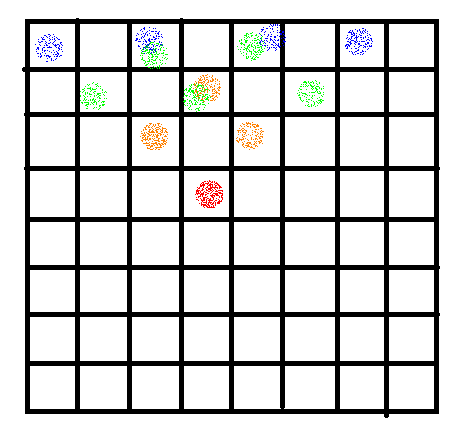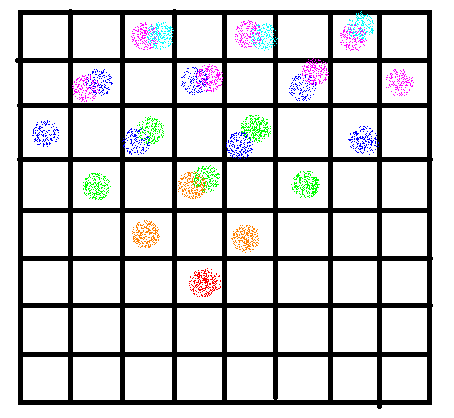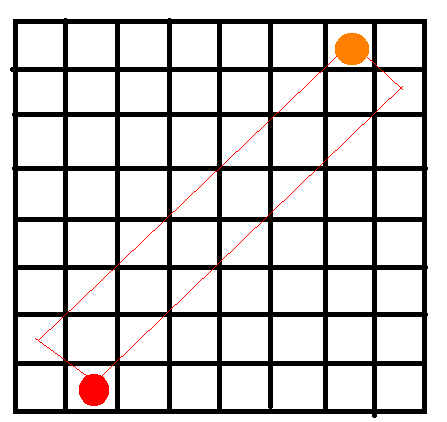# 题解# 代码

#pragma GCC optimize(2)
#include <bits/stdc++.h>
#define clr(x) memset(x,0,sizeof (x))
#define For(i,a,b) for (int i=a;i<=b;i++)
#define Fod(i,b,a) for (int i=b;i>=a;i--)
using namespace std;
typedef long long LL;
LL x=0,f=0;
char ch=getchar();
while (!isdigit(ch))
f|=ch=='-',ch=getchar();
while (isdigit(ch))
x=(x<<1)+(x<<3)+(ch^48),ch=getchar();
return f?-x:x;
}
const int N=100005,mod=1e9+7;
int n,k;
int fa[N],d[N];
int getf(int x){
if (fa[x]==x)
return x;
int f=getf(fa[x]);
d[x]^=d[fa[x]];
return fa[x]=f;
}
int Pow(int x,int y){
int ans=1;
for (;y;y>>=1,x=(LL)x*x%mod)
if (y&1)
ans=(LL)ans*x%mod;
return ans;
}
int main(){
For(i,0,n)
fa[i]=i;
while (k--){
char s;
scanf("%s",s);
z=s=='x'?0:1;
int L=y-(x-1);
if (L<1)
L=1+(1-L);
int R=y+(x-1);
if (R>n)
R=n-(R-n);
L=max(0,L-2);
if (getf(L)!=getf(R))
d[fa[L]]=z^d[L]^d[R],fa[fa[L]]=fa[R];
else if (d[L]^d[R]^z)
return puts("0"),0;
}
int ans=-1;
For(i,0,n)
if (fa[i]==i)
ans++;
if (ans==-1)
assert(0);
cout<<Pow(2,ans)<<endl;
return 0;
}


posted @ 2019-03-02 15:01  -zhouzhendong-  阅读(...)  评论(...编辑  收藏